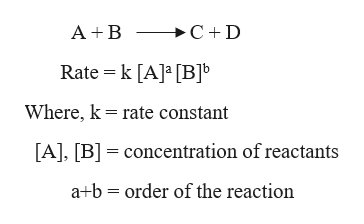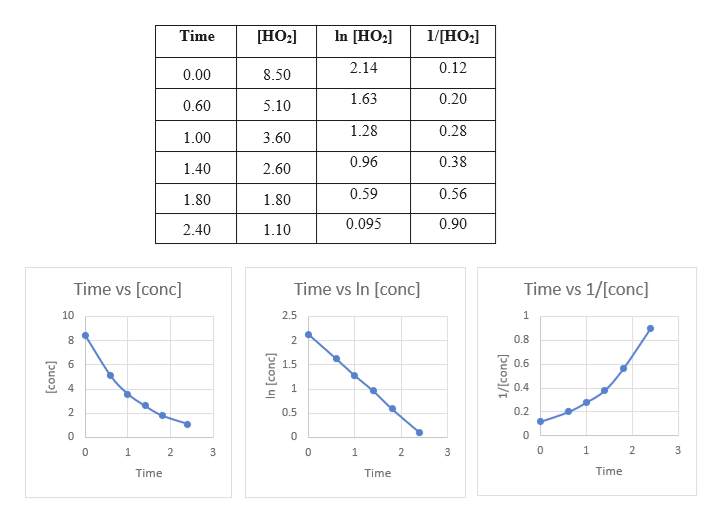Atmospheric chemistry involves highly reactive, odd-electron molecules such as the hydroperoxyl radical HO2, which decomposes into H2O2 and O2. The following data was obtained at 298 K. Time (μμs)[HO2] (μμM)0.008.500.605.101.003.601.402.601.801.802.401.10  Determine the rate law for the reaction. Do not add multiplication symbols to your answer.Rate= Determine the value of the rate constant at 298 K.     μs-1

Question
Atmospheric chemistry involves highly reactive, odd-electron molecules such as the hydroperoxyl radical HO2, which decomposes into H2O2 and O2. The following data was obtained at 298 K.

 Time (μμs) [HO2] (μμM) 0.00 8.50 0.60 5.10 1.00 3.60 1.40 2.60 1.80 1.80 2.40 1.10

Determine the rate law for the reaction. Do not add multiplication symbols to your answer.

Rate=

Determine the value of the rate constant at 298 K.
μs-1
Step 1

The rate law of a reaction is expressed as:help_outlineImage Transcriptionclose>C+ D A B Rate k [A]a [B]b Where, k rate constant [A], [B] concentration of reactants a b order of the reaction fullscreen
Step 2

Firstly, we need to find the order of the reaction. This can be done by plotting graphs of time versus concentration. If the time vs [conc] gives a straight line it will be zero order reaction.  If the time vs ln[conc] gives a straight line it will be first order reaction. If the time vs 1/[conc] gives a straight line it will be second order reaction.help_outlineImage Transcriptionclose[HO2 In [HO2 1/[HO2 Time [НO:] 2.14 0.12 8.50 0.00 0.20 1.63 0.60 5.10 0.28 1.28 1.00 3.60 0.96 0.38 1.40 2.60 0.59 0.56 1.80 1.80 0.90 0.095 2.40 1.10 Time vs [conc Time vs In [conc] Time vs 1/[conc] 10 2.5 1 0.8 0.6 6 1.5 4 0.4 1 0.2 2 0.5 0 1 2 3 1 2 3 1 2 3 Time Time Time [conc] In [conc] en 1/[conc] 생 명:명 fullscreen
Step 3

Since, time vs ln [ HO2] gives a straight line. Therefore, the reaction is first order.

The rat...

Want to see the full answer?

See Solution

Want to see this answer and more?

Our solutions are written by experts, many with advanced degrees, and available 24/7

See Solution
Tagged in

Chemical Kinetics